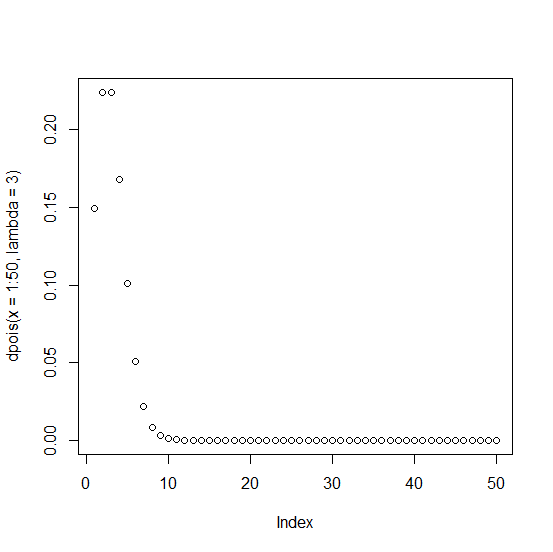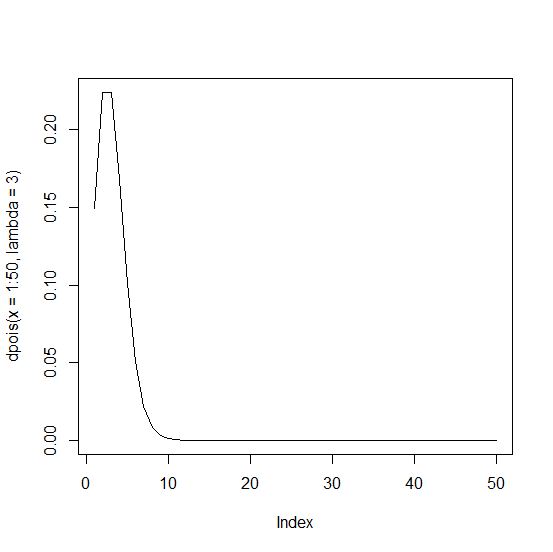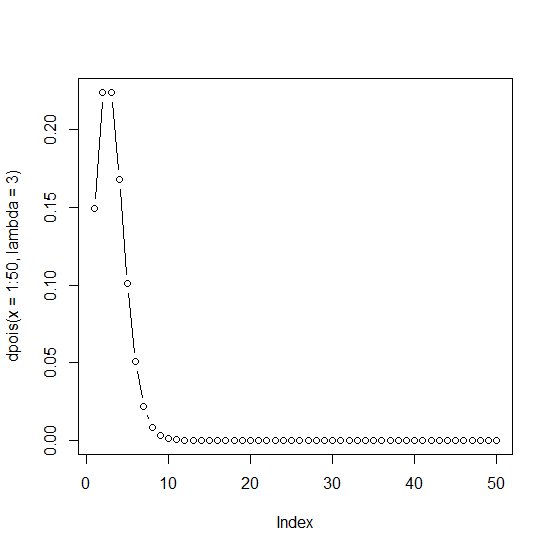# How to create a plot of Poisson distribution in R?

R ProgrammingServer Side ProgrammingProgramming

The Poisson distribution is a discrete distribution that has only one parameter named as lambda and it is the rate parameter. The rate parameter is defined as the number of events that occur in a fixed time interval. To create a plot of Poisson distribution in R, we can use the plot function with the density of the Poisson distribution using dpois function.

## Example

Live Demo

plot(dpois(x=1:50,lambda=3))

## Output## Example

Live Demo

plot(dpois(x=1:50,lambda=3),type="l")

## Output## Example

Live Demo

plot(dpois(x=1:50,lambda=3),type="b")

## Output